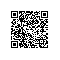# C语言内存泄露检测--Memwatch

#define fc_malloc(size) fc_malloc_imp(size,__FILE__,__LINE__)

#define fc_free(ptr) fc_free_imp(ptr)

typedef struct mem_log{
char* file;
int   line;
}memlog;
#ifdef _LOGGER
static list g_mem_list
#endif // _LOGGER
void init_module()
{
#ifdef _LOGGER
list_init(&g_mem_list);
#endif
}

void uninit_module()
{
#ifdef _LOGGER
list_for_each(g_mem_list,v)
{
memlog* iterm=(memlog*)LIST_VALUE(v);
free(iterm);
}
list_clear(&g_mem_list);

#endif // _LOGGER

}

void* fc_malloc_imp(size_t size,char* file,int line)
{
void* ptr=malloc(size);

#ifdef _LOGGER

if(ptr!=NULL)
{
memlog* iterm = (memlog*)malloc(sizeof(memlog));
iterm->file=file;
iterm->line=line;
list_insert(&g_mem_list,iterm);
}
#endif // _LOGGER
return ptr;
}
void fc_free_imp(void* ptr)
{
#ifdef _LOGGER
if(ptr!=NULL)
{
list_erase_by_addr(&g_mem_list,ptr);//in this funciton we need free the memlog iterm memory.
}

#endif // _LOGGER
free(ptr);
}使用钉钉扫一扫加入圈子
+ 订阅